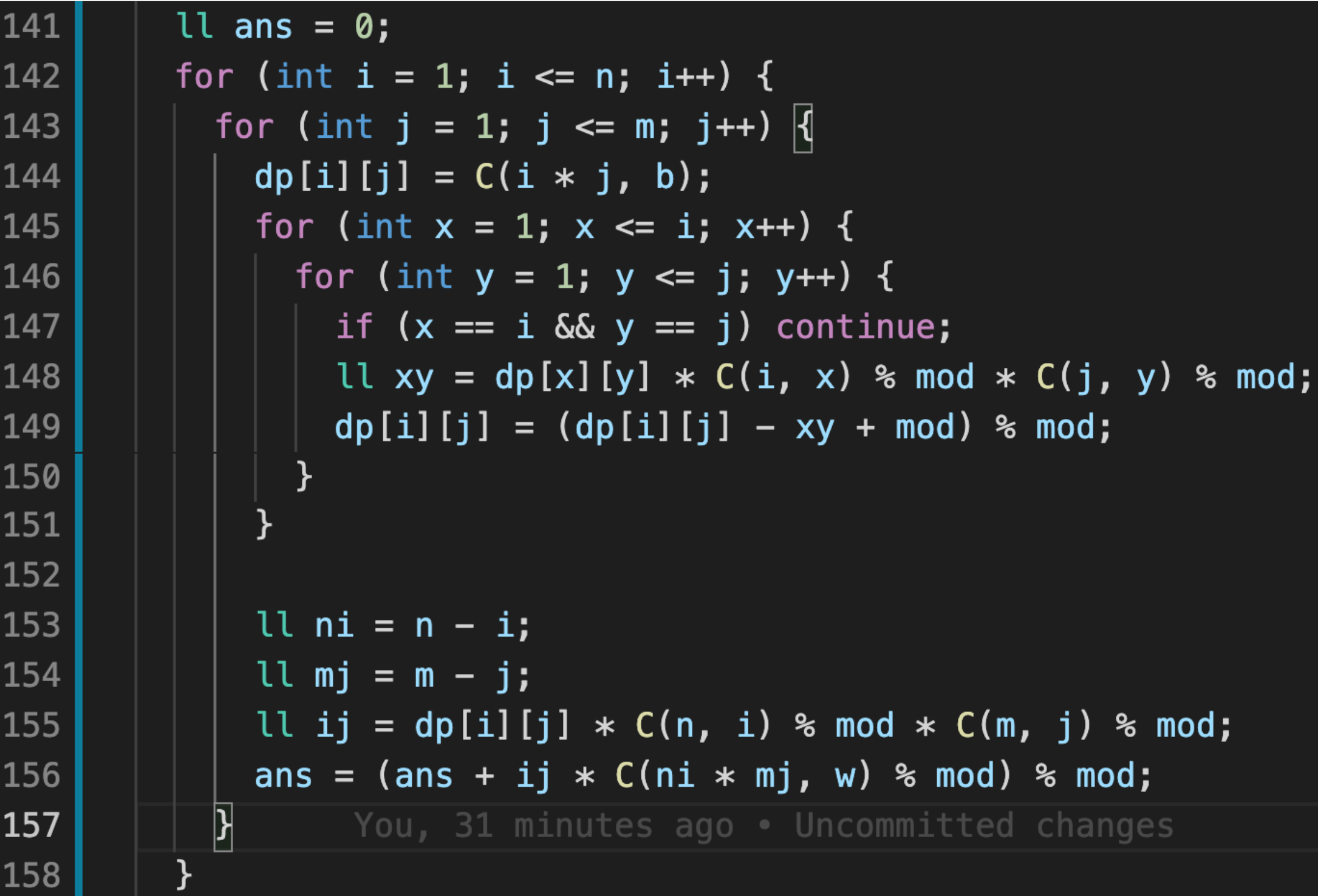# atcoder abc 第 242 场算法比赛

## A - T-shirt

`A+1` 名到第 B 名会等概率抽奖的方式发 C 个文化衫。

## B - Minimize Ordering

``````f(n, x) = f(n-1, x-1) + f(n-1, x) + f(n-1, x+1)
``````

## D - ABC Transform

``````A → BC
B → CA
C → AB
``````

``````T <= 10^18
K <= 10^18
``````

## E - (∀x∀)

``````f(x, y) = a * 26 ^ b + f(x+1, y-1)
a 为小于 str[x] 的字母个数
b = (y - x) / 2
``````

## F - Black and White Rooks

``````C(n, xb) * C(m, yb) // 黑棋的矩阵个数
* F(xb, yb, B) // 一个矩阵的方案数
* C(n-xb, xw) * C(m-yb, yw)  // 白棋的矩阵个数
* F(xw, yw, W) // 一个矩阵的方案数
``````

``````1 <= xw <= n-xb
1 <= yw <= m-yb
sum(C(n-xb, xw) * C(m-yb, yw)  * F(xw, yw, W))
= C((n-xb) * (m-yb), W)
= f((n-xb), (m-yb), W)
``````

`f(N, M, w)`的几何意义是在 `N``M` 列里面，任意摆放 W 个棋子的方案数。

F 函数是每行每列至少一个，可以理解为填充满的矩阵。
f 函数是任意摆放，没有限制，可以理解为填充满的矩阵加若干空行空列。

``````F(xb, yb, B) = f(xb, yb, B) - 不满足的情况

``````## G - Range Pairing Query

Q 个询问的复杂度就是 `O(Q N)`， 必然会超时。

``````f(l, r)
= f(l - 1, r) - F(l - 1)
= f(l + 1, r) + F(l)
= f(l, r - 1) + F(r)
= f(l, r + 1) - F(r + 1)
``````

《完》

-EOF-

## 关注公众号,接收最新消息

1.